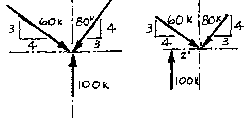## Lecture 7Example Problem

Equations of Equilibrium
Given:
two force systems as shown above.
Determine:
whether or not equilibrium has been satisfied.Solution:
In order for a system to be in equilibrium, it must satisfy all three equations of equilibrium,
Sum Fx = 0, Sum Fy = 0 and Sum M = 0.

Begin with the sum of the forces equations. The simplest way to solve these force systems would be to break the diagonal forces into their component pars. From observation, each diagonal is the "5" side of a 3-4-5 triangle. Therefore, the side marked " 3" has a value of 3/5 of the value of the diagonal and the side marked "4" is equal to 4/5 the value of the diagonal.

Now, using the components, solve for the sum of forces equations.

Sum Fx = 4/5 (60k) - 3/5 (80k) = 48 - 48 = 0

Sum Fy = 100k - 3/5 (60) - 4/5 (80) = 100 - 36 - 64 = 0

Both systems satisfy the sum of forces equations for equilibrium.

Now solve for the sum of moments equation. The system on the left is in moment equilibrium because it is a concurrent force system. Take the sum of the moments at their point of intersection. For each force, the moment arm is equal to zero. Once equil ibrium has been established using this single point, the sum of the moments for that force system will be zero for any point on that plane.

The force system on the right is not in moment equilibrium. Taking the sum of moments around the same point as before, the moment arm of the two diagonal forces are zero, but the 100# force will cause a clockwise rotation. This system cannot be p ut into equilibrium with a single force because that would disrupt the sum of forces equations. The easiest solution would be an applied moment, equal in magnitude to that caused by the 100# force, but opposite in sense.